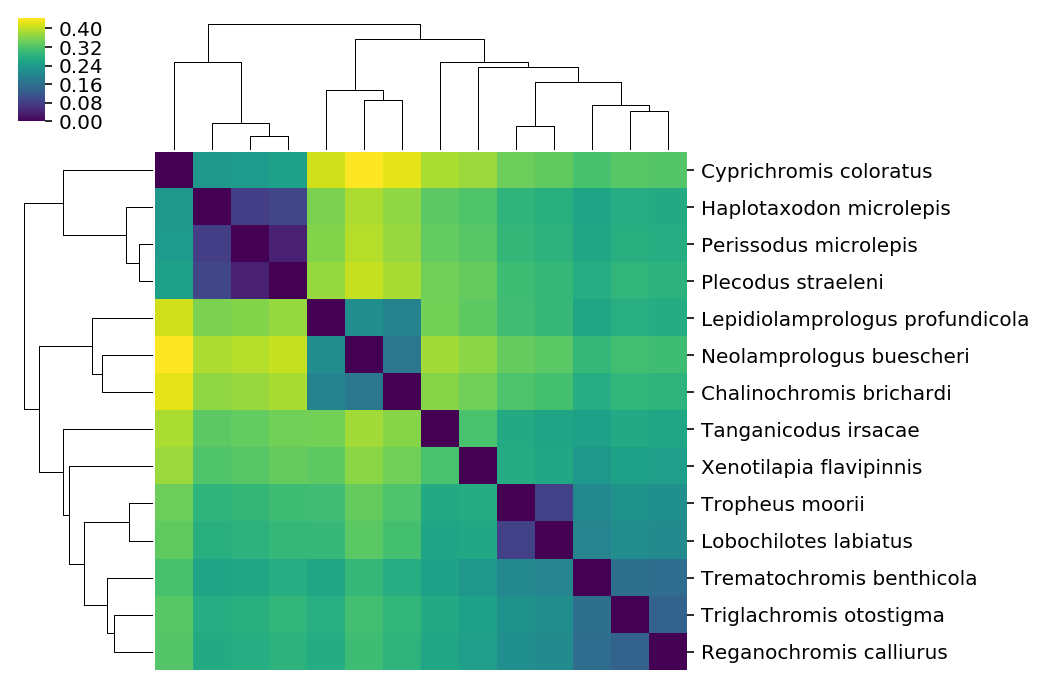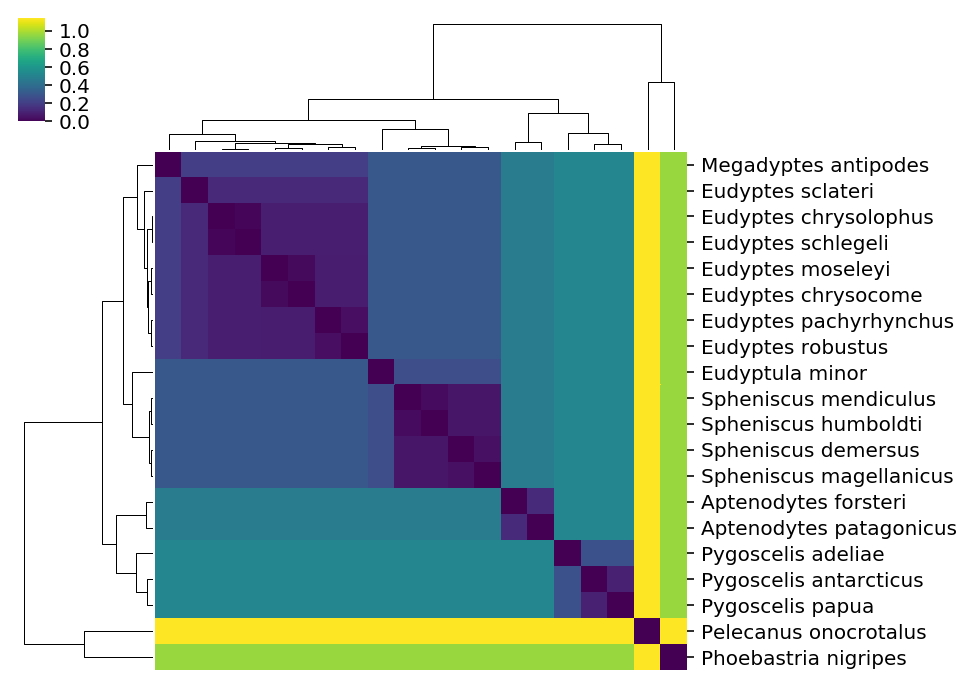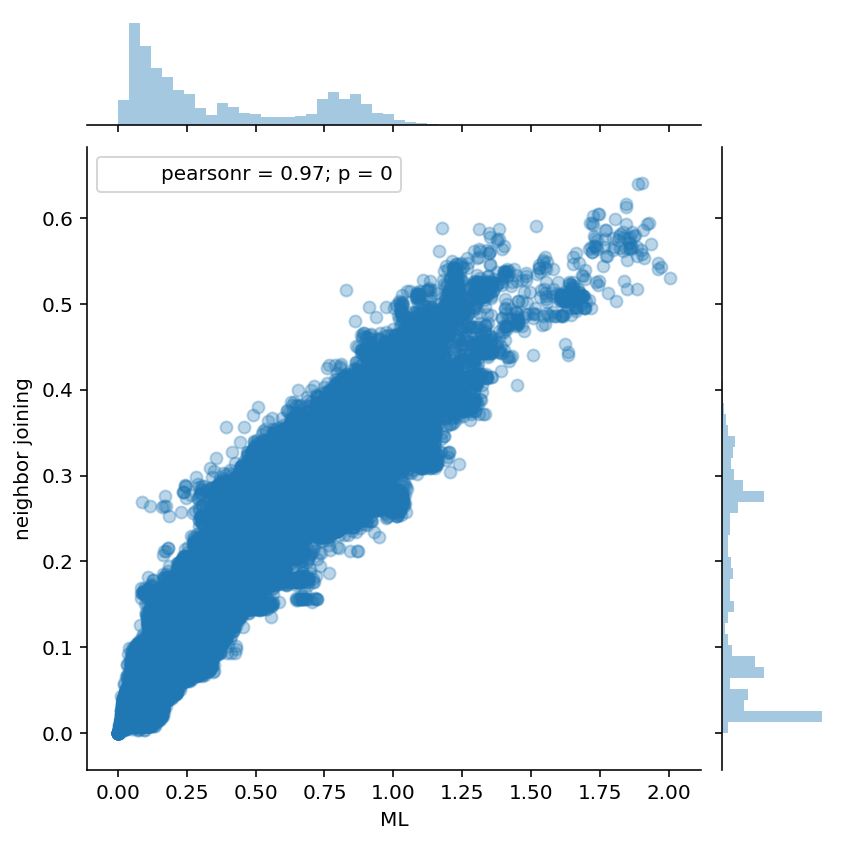There are a lot of packages for working with and manipulating phylogenetic trees using python. Rather than compete with packages like dendropy and ete3 on the basis of features, SuchTree does one thing well -- its memory usage and algorithmic complexity linearly with the number of taxa in your tree. If you need to work with very large trees, this is very helpful.

Let's have a look at some useful things you can do with trees using the SuchTree class. First, let's get our modules loaded. I'm going to suppress some warnings

To run this notebook on an Ubuntu host, you will need the following system packages installed for ete3 to work :

• python3-pyqt4.qtopengl
• python3-pyqt5.qtopengl

and python packages :

• SuchTree
• pandas
• cython
• scipy
• numpy
• matplotlib
• seaborn
• fastcluster
• dendropy
• ete3

and obviously you'll want jupyter installed so you can run the notebook server. The Internet is full of opinions about how to set up your python environment. You should find one that works for you, but this guide is as good as any to get you started.

I'm going to start off by loading the required packages and suppressing some warnings that should be fixed in the next stable release of seaborn.

I'm going to assume that you are running this notebook out of a local copy of the SuchTree repository for any local file paths.

In :
%pylab inline
%config InlineBackend.figure_format='retina'

import seaborn
import pandas
from scipy.cluster.hierarchy import ClusterWarning

warnings.simplefilter( 'ignore', UserWarning )
warnings.simplefilter( 'ignore', FutureWarning )

Populating the interactive namespace from numpy and matplotlib


Let's have a look at some example data. Here is a tree of cichlid fishes from my dissertation :

In :
from ete3 import Tree, TreeStyle, NodeStyle, TextFace
from numpy import linspace

ts = TreeStyle()
ts.mode = 'r'
ts.show_leaf_name = True
ts.branch_vertical_margin = 2
ts.scale = 1000
ts.show_leaf_name = False
ts.show_scale = False

nstyle = NodeStyle()
nstyle['size'] = 0

ete_tree = Tree( 'data/bigtrees/host.tree' )

for node in ete_tree.traverse() :
node.set_style(nstyle)
if node.is_leaf :
tf = TextFace( node.name.replace('_',' ').replace('\'','') )
tf.fsize = 10
tf.hz_align = 100

ete_tree.render("%%inline", w=120, units="mm", tree_style=ts)

Out:Loading tree data into SuchTree is pretty simple -- just give it a path to a valid Newick file. Under the hood, SuchTree uses dendropy for parsing. I figured it was better to introduce another dependency than to inflict yet another Newick parser on the world (the Newick file format has some slight ambiguities that can lead to annoying incompatibilities).

In :
T = SuchTree( 'data/bigtrees/host.tree' )


The SuchTree object has a dictionary called leafs that maps leaf names onto their node ids. We'll make extensive use of this as we put the utility through its paces.

In :
T.leafs

Out:
{'Tropheus_moorii': 0,
'Lobochilotes_labiatus': 2,
'Tanganicodus_irsacae': 4,
'Cyprichromis_coloratus': 6,
'Haplotaxodon_microlepis': 8,
'Perissodus_microlepis': 10,
'Plecodus_straeleni': 12,
'Xenotilapia_flavipinnis': 14,
'Triglachromis_otostigma': 16,
'Reganochromis_calliurus': 18,
'Trematochromis_benthicola': 20,
'Lepidiolamprologus_profundicola': 22,
'Neolamprologus_buescheri': 24,
'Chalinochromis_brichardi': 26}

### Calculating distances¶

SuchTree has two ways to calculate distances; one pair a time, or in batches. Batches are more efficient because it does each calculation without the interpreter's overhead.

Here's how to measure distances one at a time :

In :
import random

a = random.choice( list( T.leafs.values() ) )
b = random.choice( list( T.leafs.values() ) )

d = T.distance( a, b )

print( 'taxon 1  : %d' % a )
print( 'taxon 2  : %d' % b )
print( 'distance : %f' % d )

taxon 1  : 6
taxon 2  : 24
distance : 0.446084


The distance() function will accept either node ids (which are integers), or taxon names (which are strings).

In :
a = random.choice( list( T.leafs.keys() ) )
b = random.choice( list( T.leafs.keys() ) )

d = T.distance( a, b )

print( 'taxon 1  : %s' % a )
print( 'taxon 2  : %s' % b )
print( 'distance : %f' % d )

taxon 1  : Lepidiolamprologus_profundicola
taxon 2  : Reganochromis_calliurus
distance : 0.274808


You can loop over all of the distances one at a time to construct a distance matrix...

In :
D1 = zeros( ( len(T.leafs),len(T.leafs) ) )
for i,a in enumerate(T.leafs.values()) :
for j,b in enumerate( T.leafs.values() ) :
D1[i,j] = T.distance( a, b )


Let's look at the distance matrix using a nice seaborn clustermap plot.

It's worth noting that seaborn is using scipy's cluster.hierarchy functions to build those dendrograms from the distance matrix. They aren't going to have exactly the same topology as the input tree, which was build with RAxML.

In :
df = pandas.DataFrame( D1, index=[ i.replace('_',' ') for i in T.leafs.keys() ] )
seaborn.clustermap( df, xticklabels=False, cmap='viridis', figsize=(6,6) )

Out:
<seaborn.matrix.ClusterGrid at 0x7fa057c9a080>To calculate the distances in a batch, we can use the distances() function, which takes an $n \times 2$ array of node ids (make sure your array is representing them as integers).

In :
D2_list = []
for i,a in enumerate(T.leafs.values()) :
for j,b in enumerate( T.leafs.values() ) :
D2_list.append( ( a, b ) )
D2_array = array( D2_list )

print( D2_array.shape )
print( D2_array[:5] )

(196, 2)
[[0 0]
[0 2]
[0 4]
[0 6]
[0 8]]

In :
D2 = T.distances( D2_array )

D2 = D2.reshape( ( len(T.leafs), len(T.leafs) ) )


We should get the same distance matrix and clustermap as before.

In :
df = pandas.DataFrame( D2, index=[ i.replace('_',' ') for i in T.leafs.keys() ] )
seaborn.clustermap( df, xticklabels=False, cmap='viridis', figsize=(6,6) )

Out:
<seaborn.matrix.ClusterGrid at 0x7fa04c09bf28>If you want to use taxon names instead, distances_by_name() accepts an $n \times 2$ list of tuples of taxon names, and looks up the node ids for you.

SuchTree can also import data from the internets. Here is the distance matrix for the penguins, from the Global Phylogeny of Birds (I've copied some of their data into a Gist post because their data repository forbids programatic queries).

In :
T3 = SuchTree( 'https://git.io/flbcP' )

D3_list = []
for i,a in enumerate(T3.leafs.values()) :
for j,b in enumerate( T3.leafs.values() ) :
D3_list.append( ( a, b ) )
D3_array = array( D3_list )
D3 = T3.distances( D3_array )
D3 = D3.reshape( ( len(T3.leafs), len(T3.leafs) ) )

df = pandas.DataFrame( D3, index=[ i.replace('_',' ') for i in T3.leafs.keys() ] )
seaborn.clustermap( df, xticklabels=False, cmap='viridis', figsize=(6,6) )

Out:
<seaborn.matrix.ClusterGrid at 0x7fa0440a9400>### Comparing the topologies of two large trees¶

So far, we haven't done anything you couldn't do with other phylogenetics packages. SuchTree really shines when you have to do a lot of distance calculations on very large trees.

Here, we use SuchTree to compare the topology of a two trees containing the taxa but constructed with different methods (FastTree and neighbor joining). One million random pairs are sampled from each tree, and the distances compared.

In :
T1 = SuchTree( 'https://git.io/flbcM' )
T2 = SuchTree( 'https://git.io/flbcH' )

print( 'nodes : %d, leafs : %d' % ( T1.length, len(T1.leafs) ) )
print( 'nodes : %d, leafs : %d' % ( T2.length, len(T2.leafs) ) )

nodes : 108653, leafs : 54327
nodes : 108653, leafs : 54327

In :
N = 1000000

v = list( T1.leafs.keys() )

pairs = []
for i in range(N) :
pairs.append( ( random.choice( v ), random.choice( v ) ) )

%time D1, D2 = T1.distances_by_name( pairs ), T2.distances_by_name( pairs )

CPU times: user 15.6 s, sys: 43.2 ms, total: 15.6 s
Wall time: 15.6 s


The distances() function, which uses node ids rather than taxa names, would be a little bit faster. However, because the trees have different topologies, the taxa have different node ids in each tree. SuchTree's distances_by_name() function untangles the leaf name to leaf node id mappings for you.

In :
df = pandas.DataFrame( { 'ML' : D1, 'neighbor joining' : D2 } )

seaborn.jointplot( 'ML', 'neighbor joining', data=df, alpha=0.3, size=6 )

Out:
<seaborn.axisgrid.JointGrid at 0x7fa02a460668>In :
from scipy.stats import spearmanr, kendalltau, pearsonr

print( 'Spearman\'s rs : %0.3f' % spearmanr(  D1, D2 ) )
print( 'Kendall\'s tau : %0.3f' % kendalltau( D1, D2 ) )
print( 'Pearson\'s r   : %0.3f' % pearsonr(   D1, D2 ) )

Spearman's rs : 0.961
Kendall's tau : 0.824
Pearson's r   : 0.969


Another advantage of SuchTree's support for performing batches of distance calculations is that these calculations can run outside of Python's global interpreter lock. This makes it possible to parallelize with Threads. Python's Thread has less overhead than the multiprocessing package's Process, and Thread objects can access the same memory.

SuchTree intentionally does not allow the user to alter trees once they are created, and so distance calculations are always thread safe. This makes it possible to use only one instance of a tree for all threads, which ought to give you the best chance of keeping it within L3 cache.

First, let's create a Cython function that calls SuchTree outside of the GIL.

In :
%load_ext Cython

In :
%%cython
import cython

def correlation( double[:] x, double[:] y ) :
return _correlation( x, y )

@cython.boundscheck(False)
cdef double _correlation( double[:] x, double[:] y ) nogil :
cdef int n = x.shape
cdef int i = 0
cdef double r = 0.0
cdef double xbar = 0.0
cdef double ybar = 0.0
cdef double sx = 0.0
cdef double sy = 0.0

for i in xrange(n) :
xbar += x[i]
ybar += y[i]
xbar /= n
ybar /= n

# compute standard deviation of x and y
for i in xrange(n) :
sx += (x[i] - xbar) * (x[i] - xbar)
sy += (y[i] - ybar) * (y[i] - ybar)

sx = ( sx / n )**(0.5)
sy = ( sy / n )**(0.5)

# compute r, the correlation coefficient between the two arrays
for i in xrange(n) :
r += (((x[i] - xbar)/sx) * ((y[i] - ybar)/sy));
r /= (n);
return r


Let's set up two queues, one for uncompleted tasks, which we'll call work_q, and one for finished results, which we'll call done_q. The worker threads will pop tasks from the work_q, do some work, and push the results into the done_q.

Let's also push sentinels at the end of the work_q so the workers have a convenient way to know when to shut down.

In :
from threading import Thread
from queue import Queue

n = 4  # number of threads
m = 12 # number of work units

work_q = Queue()
done_q = Queue()

v = list( T1.leafs.keys() )

print( 'building work blocks...' )
for i in range( m ) :
pairs = []
for i in range( 100000 ) :
pairs.append( ( T1.leafs[ random.choice( v ) ],
T2.leafs[ random.choice( v ) ] ) )
work_q.put( array( pairs, dtype=int ) )

for i in range( n ) :
work_q.put( 'STOP' ) # sentinel elements, one for each worker

building work blocks...


The worker process takes the two queues as arguments, and returns True when it completes successfully.

Note that we're not using distances_by_name() because it requires a call to Python's dictionary object, which requires the GIL. Instead, we have looked up the node id for each taxon when we created the tasks we pushed onto work_q.

In :
def worker( work_q, done_q ) :
print( 'starting worker...' )
for task in iter( work_q.get, 'STOP' ) :
r = correlation( D1, D2 )
done_q.put( r )
print( 'stopping worker...' )
return True


Now we can set up our thread pool, run it and print the results the threads pushed onto done_q.

In :
threads = []

for i in range( n ) :

done_q.put( 'STOP' )

for r in iter( done_q.get, 'STOP' ) :
print( r )

starting worker...
starting worker...
starting worker...
starting worker...
stopping worker...
stopping worker...
stopping worker...
stopping worker...
0.7858568333588076
0.7826590873988822
0.7844420298785083
0.7838968592880762
0.7832616169597755
0.7810781477882289
0.7823715835475968
0.7832743632827825
0.7862756657001954
0.7851411483753257
0.7828403864806949
0.7833013061598763


Threaded algorithms are difficult to implement, and this is a very minimalist approach. It's really only good enough to demonstrate that you can call SuchTree from your worker threads without tripping the interpreter lock.

If you need this capability, you will probably want to study the documentation carefully.